/ lrslibrary Public

Low-Rank and Sparse Tools for Background Modeling and Subtraction in Videos

# andrewssobral/lrslibrary

Switch branches/tags
Nothing to show

A tag already exists with the provided branch name. Many Git commands accept both tag and branch names, so creating this branch may cause unexpected behavior. Are you sure you want to create this branch?

## Latest commit

`06d4573`

## Files

Failed to load latest commit information.Last Page Update: 29/07/2022, Previous Page Update: 07/03/2020

## LRSLibrary

Low-Rank and Sparse tools for Background Modeling and Subtraction in Videos.

The LRSLibrary provides a collection of low-rank and sparse decomposition algorithms in MATLAB. The library was designed for moving object detection in videos, but it can be also used for other computer vision and machine learning problems (for more information, please see here and here). Currently the LRSLibrary offers more than 100 algorithms based on matrix and tensor methods. The LRSLibrary was tested successfully in several MATLAB versions (e.g. R2014, R2015, R2016, R2017, on both x86 and x64 versions). It requires minimum R2014b.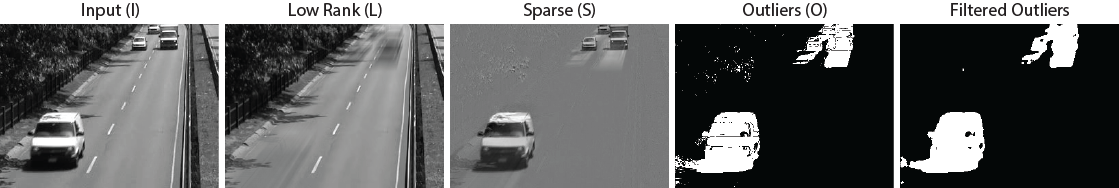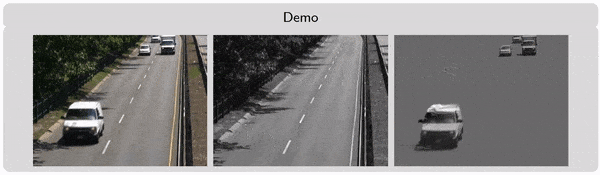``````Presentation about Matrix and Tensor Tools for Computer Vision
http://www.slideshare.net/andrewssobral/matrix-and-tensor-tools-for-computer-vision

MTT: Matlab Tensor Tools for Computer Vision
https://github.com/andrewssobral/mtt

IMTSL: Incremental and Multi-feature Tensor Subspace Learning
https://github.com/andrewssobral/imtsl
``````

## Citation

If you use this library for your publications, please cite it as:

``````@incollection{lrslibrary2015,
author    = {Sobral, Andrews and Bouwmans, Thierry and Zahzah, El-hadi},
title     = {LRSLibrary: Low-Rank and Sparse tools for Background Modeling and Subtraction in Videos},
booktitle = {Robust Low-Rank and Sparse Matrix Decomposition: Applications in Image and Video Processing},
publisher = {CRC Press, Taylor and Francis Group.}
year      = {2015}
}
``````

``````@article{bouwmans2015,
author    = {Bouwmans, Thierry and Sobral, Andrews and Javed, Sajid and Jung, Soon Ki and Zahzah, El-hadi},
title     = {Decomposition into Low-rank plus Additive Matrices for Background/Foreground Separation: {A} Review for a Comparative Evaluation with a Large-Scale Dataset},
journal   = {CoRR},
volume    = {abs/1511.01245}
year      = {2015},
url       = {http://arxiv.org/abs/1511.01245}
}
``````

## Stargazers over time## Install

Just do the following steps:

• First, clone the repository:

`\$ git clone --recursive https://github.com/andrewssobral/lrslibrary.git`

• Then, open your MATLAB and run the following setup script:

`>> lrs_setup`

That's all!

## GUI

The LRSLibrary provides an easy-to-use graphical user interface (GUI) for background modeling and subtraction in videos. First, run the setup script lrs_setup (or run('C:/lrslibrary/lrs_setup')), then run lrs_gui, and enjoy it!

(Click in the image to see the video)

Each algorithm is classified by its cpu time consumption with the following icons: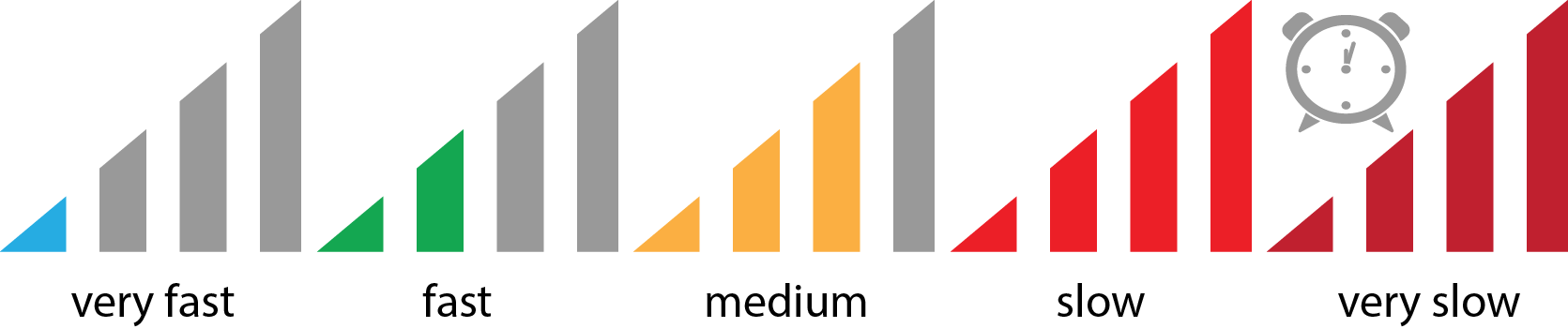The algorithms were grouped in eight categories: RPCA for Robust PCA, ST for Subspace Tracking, MC for Matrix Completion, TTD for Three-Term Decomposition, LRR for Low-Rank Representation, NMF for Non-negative Matrix Factorization, NTF for Non-negative Tensor Factorization, or TD for standard Tensor Decomposition.

## Usage example

For complete details and examples, please see the demo.m file.

```%% First run the setup script
lrs_setup; % or run('C:/lrslibrary/lrs_setup')

input_avi = fullfile(lrs_conf.lrs_dir,'dataset','demo.avi');
output_avi = fullfile(lrs_conf.lrs_dir,'output','output.avi');

%% Processing videos
%
% Robust PCA
process_video('RPCA', 'FPCP', input_avi, output_avi);
% Subspace Tracking
process_video('ST', 'GRASTA', input_avi, output_avi);
% Matrix Completion
process_video('MC', 'GROUSE', input_avi, output_avi);
% Low Rank Recovery
% Three-Term Decomposition
process_video('TTD', '3WD', input_avi, output_avi);
% Non-Negative Matrix Factorization
process_video('NMF', 'ManhNMF', input_avi, output_avi);
% Non-Negative Tensor Factorization
process_video('NTF', 'bcuNCP', input_avi, output_avi);
% Tensor Decomposition
process_video('TD', 'Tucker-ALS', input_avi, output_avi);

%% Processing matrices and tensors
%
V = im2double(imgdb{100});
show_3dvideo(V);

%% Matrix-based algorithms
%
[M,m,n,p] = convert_video3d_to_2d(V);
show_2dvideo(M,m,n);

% Robust PCA
out = run_algorithm('RPCA', 'FPCP', M);
% Subspace Tracking
out = run_algorithm('ST', 'GRASTA', M);
% Matrix Completion
obs = 0.5; % Percentual of observed entries [0...1]
[params.Idx, params.Omega] = subsampling(M, obs);
out = run_algorithm('MC', 'GROUSE', M, params);
% Low Rank Recovery
% Three-Term Decomposition
out = run_algorithm('TTD', '3WD', M);
% Non-Negative Matrix Factorization
out = run_algorithm('NMF', 'ManhNMF', M);

% Show results
show_results(M,out.L,out.S,out.O,p,m,n);

%% Tensor-based algorithms
T = tensor(V);

% Non-Negative Tensor Factorization
out = run_algorithm('NTF', 'bcuNCP', T);
% Tensor Decomposition
out = run_algorithm('TD', 'Tucker-ALS', T);

% Show results
show_3dtensors(T,out.L,out.S,out.O);```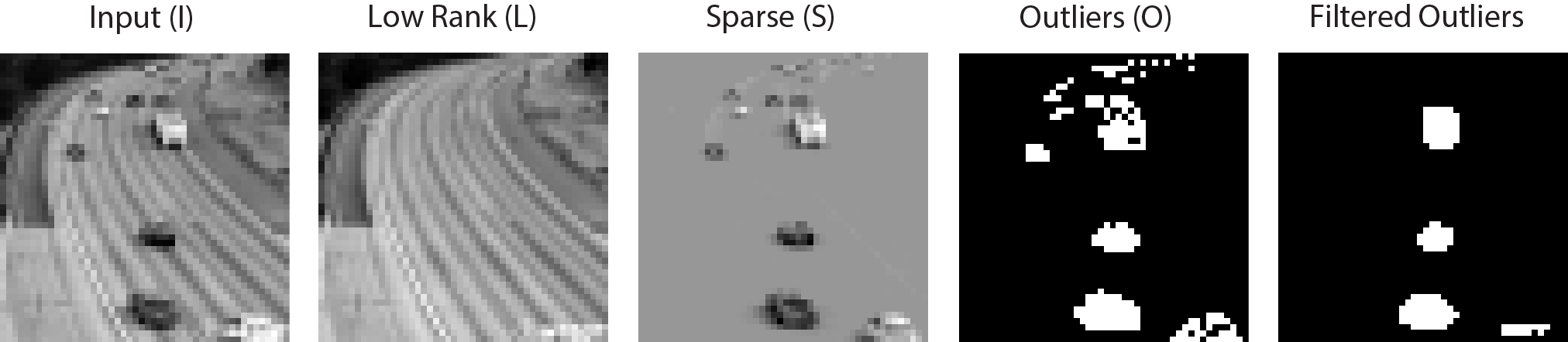## CPU time consumption

The figure below shows the average CPU time consumption and the speed classification of each algorithm to decompose a 2304x51 matrix or 48x48x51 tensor data. Both matrix and tensor data were built from dataset/demo.avi file. The experiments were performed in a Intel Core i7-3740QM CPU 2.70GHz with 16Gb of RAM running MATLAB R2013b and Windows 7 Professional SP1 64 bits.

A complete review evaluating the algorithms in many specific criterias will be published in a paper journal soon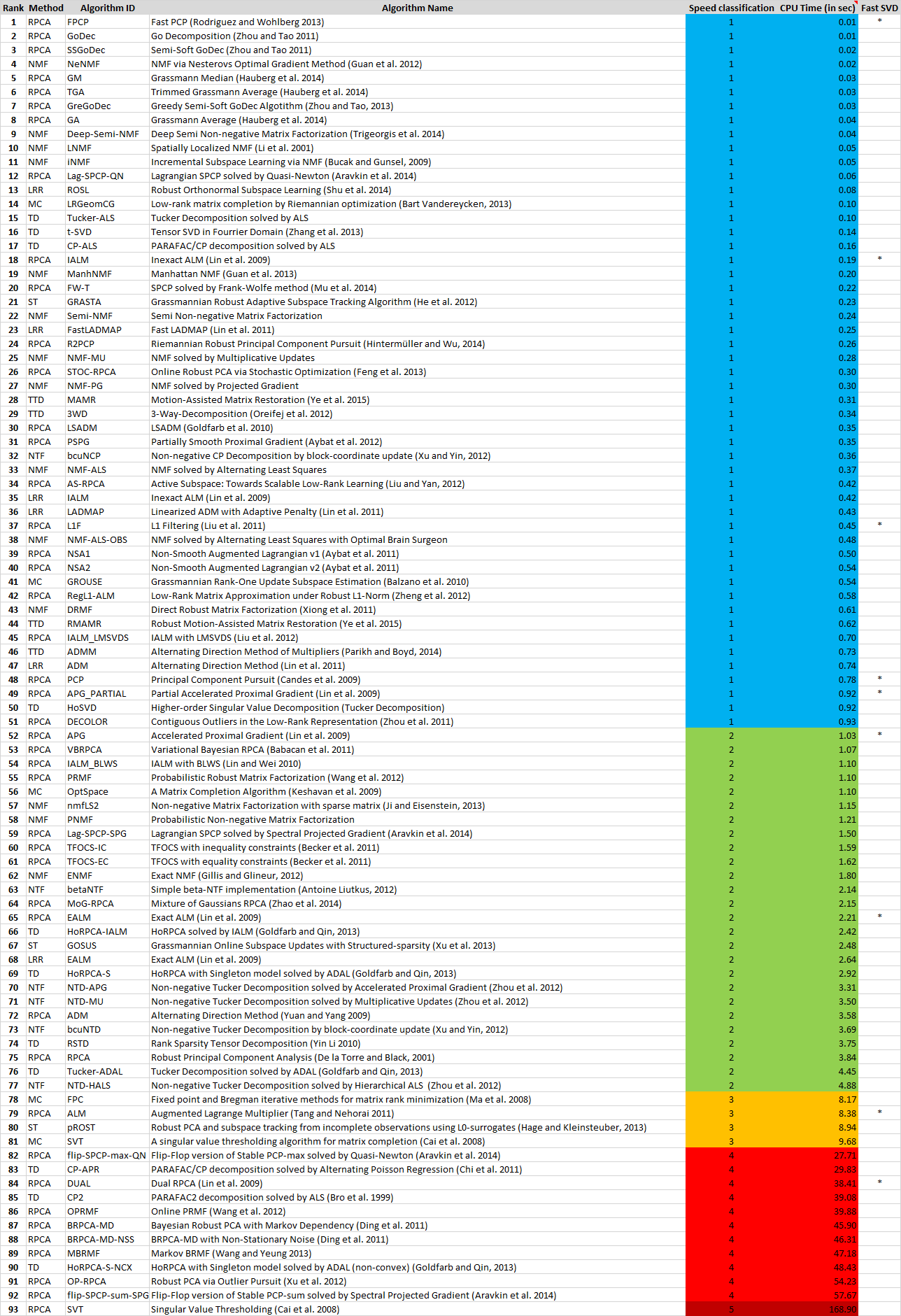The LRSLibrary has been developed by Andrews Sobral thanks especially to Thierry Bouwmans for his continued support and for collaborating on this important initiative. I'm grateful to all authors who have contributed in some way to the success of the LRSLibrary.

## How to contribute with LRSLibrary project

Everyone is invited to cooperate with the LRSLibrary project by sending to us any implementation of low-rank and sparse decomposition algorithms.

Option 1: email it to me (andrewssobral at gmail dot com).

Option 2: fork the library on GitHub, push your changes, then send me a pull request.

For the Option 2 you can follow the instructions below:

1. Create the algorithm folder in lrslibrary/algorithms/XYZ/ where "XYZ" is one of the following methods: RPCA (for Robust PCA), ST (for Subspace Tracking), MC (for Matrix Completion), LRR (for Low Rank Recovery), TTD (for Three-Term Decomposition), NMF (for Non-Negative Matrix Factorization), NTF (for Non-Negative Tensor Factorization), and TD (for Tensor Decomposition).

2. Create a run_alg.m script file to execute your algorithm, all run_alg.m files are called automatically by the lrslibrary and each of them receives a full rank matrix/tensor named "M" or "T" for a matrix-based or a tensor-based algorithm, respectively. For the matrix M, each column is a vectorized version of a gray-scale frame. The number of columns in M is the number of frames given a video, and the number of rows represents the number of pixels in the frame. For the tensor T each frontal slice represents a frame given a video.

2.1) For the matrix/tensor completion case you need to define a random sub-sampling matrix/tensor named "Idx" that indicates which elements from the input matrix/tensor (M or T) are observed (setting 1's and 0's for observed and unobserved elements, respectively).

1. The output of your run_alg.m script file are a matrix/tensor named "L" that represents the low-rank component, and a matrix/tensor named "S" that represents the sparse components.

2. The last step is to add your algorithm details in the lrs_algorithms.dat file (open it as text file): https://github.com/andrewssobral/lrslibrary/blob/master/lrs_algorithms.dat For example: XYZ | ABB | NAME_REFERENCE | SPEED_CLASS where:

• XYZ is one of the following options: RPCA, ST, MC, LRR, TTD, NMF, NTF, or TD.
• ABB abbreviation name for your method, i.e: APG for Accelerated Proximal Gradient.
• NAME_REFERENCE the full name of your method and reference, i.e: Accelerated Proximal Gradient (Lin et al. 2009).
• SPEED_CLASS represents the speed classification of your algorithm for the demo case. For this, you need to compare the CPU time consumption of your algorithm from all others following this section: https://github.com/andrewssobral/lrslibrary#cpu-time-consumption

i.e.: RPCA | FPCP | Fast PCP (Rodriguez and Wohlberg, 2013) | 1

That's all ;-)

The LRSLibrary is free and open source for academic/research purposes (non-commercial)¹.

¹ Some algorithms of the LRSLibrary are free for commercial purposes and others not. First you need to contact the authors of your desired algorithm and check with them the appropriate license.

## Problems or Questions

If you have any problems or questions, please contact the author: Andrews Sobral (andrewssobral at gmail dot com)

## Release Notes:

• Version 1.0.11: Fixed IALM with LMSVDS (Liu et al. 2012).

• Version 1.0.10: Added ReProCS (Narayanamurthy and Vaswani, 2017a) and MEDRoP (Narayanamurthy and Vaswani, 2017b). Thanks to Praneeth Narayanamurthy.

• Version 1.0.9: Fixed some issues for matrix completion methods.

• Version 1.0.8: Added PG-RMC (Cherapanamjeri et al. 2016) and fixed some small issues.

• Version 1.0.7: Code refactoring: process_matrix(), process_tensor(), run_algorithm_###() were excluded. A standard interface called run_algorithm was created. For each algorithm, there is a run_alg.m script for execution. Added 10 new algorithms: OSTD (Sobral et al. 2015), Nonconvex RPCA (Kang et al. 2015), MC_logdet (Kang et al. 2016), RPCA-GD (Yi et al. 2016), LMaFit (Wen et al. 2012), MC-NMF (Xu et al. 2011), ScGrassMC (Ngo and Saad, 2012), SVP (Meka et al. 2009), OR1MP (Wang et al. 2015), IALM-MC (Lin et al. 2009). OP-RPCA was moved from RPCA to MC category.

• Version 1.0.6: Added three new algorithms: STOC-RPCA: Online Robust PCA via Stochastic Optimization of Feng et al. (2013), MoG-RPCA: Mixture of Gaussians RPCA of Zhao et al. (2014), and OP-RPCA: Robust PCA via Outlier Pursuit of Xu et al. (2012).

• Version 1.0.5: Added three new method categories ST (Subspace Tracking), MC (Matrix Completion), and TTD (Three-Term Decomposition). Added fifteen new algorithms: 3WD - 3-Way-Decomposition of Oreifej et al. (2012), MAMR and Robust MAMR - Motion-Assisted Matrix Restoration of Ye et al. (2015), ADMM - Alternating Direction Method of Multipliers of Parikh and Boyd (2014), GreGoDec - Greedy Semi-Soft GoDec Algotithm of Zhou and Tao (2013), GRASTA (Grassmannian Robust Adaptive Subspace Tracking Algorithm) of He et al. (2012), GOSUS (Grassmannian Rank-One Update Subspace Estimation) of Balzano et al. (2010), OptSpace - A Matrix Completion Algorithm of Keshavan et al. (2009), FPC - Fixed point and Bregman iterative methods for matrix rank minimization of Ma et al. (2008), SVT - A singular value thresholding algorithm for matrix completion of Cai et al. (2008), LRGeomCG - Low-rank matrix completion by Riemannian optimization of Bart Vandereycken (2013), RPCA - Robust Principal Component Analysis of De la Torre and Black (2001), GA - Grassmann Average, GM - Grassmann Median, and TGA - Trimmed Grassmann Average of Hauberg et al. (2014). Also fixed some errors.

• Version 1.0.4: Added a setup script, and configuration file. Also fixed some errors.

• Version 1.0.3: Added three new algorithms: FW-T (Mu et al. 2014), iNMF (Bucak and Gunsel, 2009) and DRMF (Xiong et al. 2011).

• Version 1.0.2: Added four new algorithms: GOSUS (Xu et al. 2013), pROST (Hage and Kleinsteuber, 2013), RegL1-ALM (Zheng et al. 2012) and ROSL (Shu et al. 2014).

• Version 1.0.1: Added RPCA-SPCP algorithms of Aravkin et al. (2014), thanks to Professor Stephen Becker.

• Version 1.0.0: First version with 64 algorithms.

Low-Rank and Sparse Tools for Background Modeling and Subtraction in Videos

## Releases

No releases published

## Packages 0

No packages published

•
•
•